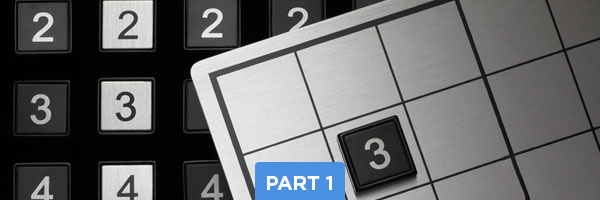# Intermediate Sudoku Solving Techniques – Part 1: Looking for Number PairsOne of the first “advanced” Sudoku solving techniques that you might want to try is to start with one number at a time (starting with the number 1) and look for instances where two of the same number appear within the same set of three squares. If you can find places where two instances of the same number already appear, that makes it easier to eliminate spaces and figure out where the remaining instance of the number needs to be placed. By focusing on just one number at a time, this technique also enables you to be methodical and stay organized in your thought process as you work through the grid.

For example, in this grid shown below, we’re starting with the number 1. In the set of three squares on the left, there are two number 1s in the central and right columns (circled in blue) and we can use this information to figure out where the number 1 needs to go within the left central square.

By process of elimination, we know that the empty space in the bottom row, center column cannot contain #1 (marked with an X) because there is already a 1 in that column (circled below). The right column was already eliminated because it is full of other numbers (outlined in blue), so that means that the only space for the number 1 is the lower left space of that square (highlighted in orange).

Now that we’ve completed that first “pair of 1s,” let’s look for another instance of two number 1s. In the central set of squares, we can see two 1s in the left and center columns (circled in blue). This shows us that in the lower-center square, there cannot be a 1 in the left column (marked with an X) and there cannot be a 1 in the right column (marked with an X – there is already a 1 in that row in the square to the left, circled in blue). This means that the 1 must be placed in the lower right space of the square, highlighted in orange.

By focusing on one number at a time, we can methodically work through the Sudoku grid.

Moving on to the number 2, let’s look for any instances where there are two #2s within a set of the same three squares.

For example, in the bottom set of squares shown below, there are two 2s, one in the top row, and one in the middle row (circled in blue). We can also see a 2 in the left column of the grid (circled in blue), so that helps us eliminate several spaces from the lower left square (marked with Xs). This means that the 2 can only be placed in the lower-right space of that square (highlighted in orange).

Moving on to the number 3, we can see two 3s in the lower set of three squares. Simple process of elimination (as described in the other examples above) indicates that the number 3 must appear in the lower left corner of the square – highlighted in orange.

Moving on the number 4, we can see a few instances of number 4s in the top set of squares (top row, bottom row – circled in blue) and also in the right set of squared (left and center columns, circled in blue). This gives us useful information to help solve the upper right square, which currently has only one number (9) placed. By looking at the existing placements of 4s, we can rule out the top row (marked with Xs), the left column (marked with Xs) and the center column (marked with Xs). This leaves only one space – the right-center space – where the number 4 can go (highlighted in orange).

This is a simple example of how you can use a more advanced Sudoku solving mindset to approach the Sudoku grid with focus and a repeatable method: look for “pairs” of numbers within the same set of squared. Focus on one number at a time. Then watch the game open up to you.